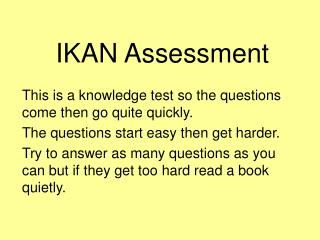DownloadDownload PresentationIKAN Assessment

# IKAN Assessment

Télécharger la présentation## IKAN Assessment

- - - - - - - - - - - - - - - - - - - - - - - - - - - E N D - - - - - - - - - - - - - - - - - - - - - - - - - - -
##### Presentation Transcript

1. IKAN Assessment This is a knowledge test so the questions come then go quite quickly. The questions start easy then get harder. Try to answer as many questions as you can but if they get too hard read a book quietly.

2. Bank One

3. Question 1 What number is one more than 79?

4. Question 2 What number is one less than 30?

5. Question 3 Write the fraction for one half.

6. Question 4 Write the fraction for one fifth.

7. Question 5 How many tens are in 80?

8. Question 6 What is the number for nine groups of ten?

9. Question 7 7 + 7 = ?

10. Question 8 Half of 18 is ..?

11. Bank Two

12. Question 1 What number is one more than 599?

13. Question 2 What number is one less than 1000?

14. 1 5 1 6 Question 3 Which fraction is bigger, or ?

15. 1 3 1 5 1 4 Question 4 Write these fractions in order of size, smallest to biggest.

16. Question 5 How many tens are in 830?

17. Question 6 What is the number for 49 groups of ten?

18. Question 7 7 + 9 = ?

19. Question 8 7 x 5 = ?

20. Bank Three

21. Question 1 What number is one more than 439 999?

22. Question 2 What number is one less than 801 000?

23. 1 3 12 4 Question 3 Which number is the same as ? 48 8 3

24. Write 4 as a fraction. 1 5 Question 4

25. Question 5 How many hundreds are in 35 600?

26. Question 6 How many tenths are in all of the number, 5.8?

27. Question 7 15 - 8 = ?

28. Question 8 6 x 7 = ?

29. Bank Four

30. Which number is the same as ? 1 3 5 5 3 2 3 12 20 4 6 Question 1

31. 1 2 4 8 50 100 8 17 3 6 Question 2 Which fraction does not equal ?

32. Question 3 Which decimal is bigger, 0.639 or 0.84?

33. Question 4 Which decimal is smaller, 2.7 or 1.987?

34. Question 5 How many hundredths are in all of 3.04?

35. Question 6 63 ÷ 9 = ?

36. Question 7 • ÷ 7 = 6? What number divided by seven gives six?

37. Question 8 Write all the factors of 81.

38. Bank Five

39. 3 4 7 10 Question 1 Which fraction is bigger, or ?

40. 3 8 1 3 Question 2 Which fraction is smaller or ?

41. Question 3 How many hundredths are in all of 6.073?

42. Question 4 What number is half-way between 4.8 and 4.7?

43. Question 5 What is the simplest fraction for 80%?

44. Question 6 What is 1.3 written as a percentage?

45. Question 7 What is the least common multiple of 6 and 9?

46. Question 8 What is the highest common factor of 36 and 48?

47. You have finished the IKAN assessment. Change your answer sheet with a partner so you can mark their answers. The next slides show the answers one by one.

48. Bank One